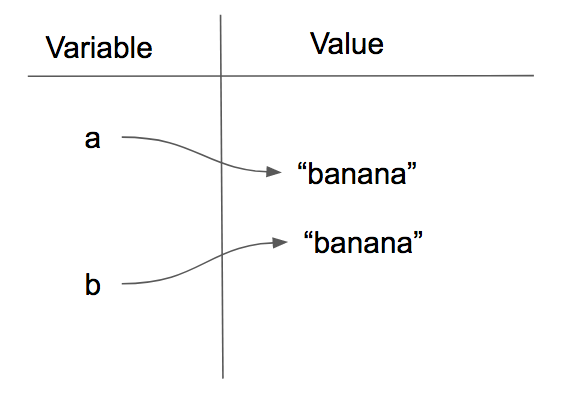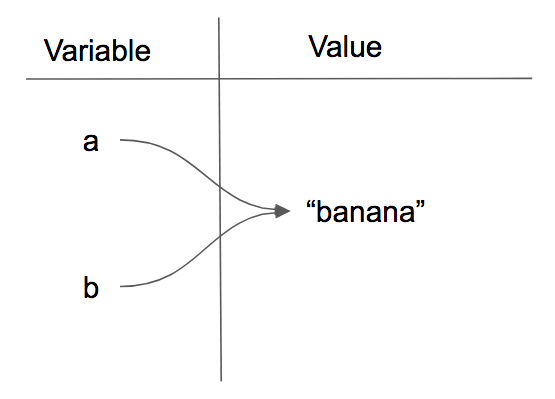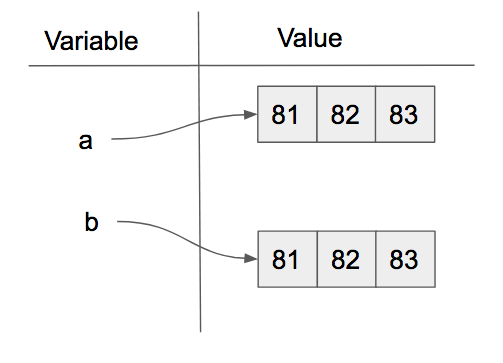# 9.4. Objects and References¶

If we execute these assignment statements,

a = "banana"
b = "banana"


we know that a and b will refer to a string with the letters "banana". But we don’t know yet whether they point to the same string.

There are two possible ways the Python interpreter could arrange its internal states:orIn one case, a and b refer to two different string objects that have the same value. In the second case, they refer to the same object. Remember that an object is something a variable can refer to.

We can test whether two names refer to the same object using the is operator. The is operator will return true if the two references are to the same object. In other words, the references are the same. Try our example from above.

The answer is True. This tells us that both a and b refer to the same object, and that it is the second of the two reference diagrams that describes the relationship. Python assigns every object a unique id and when we ask a is b what python is really doing is checking to see if id(a) == id(b).

Since strings are immutable, the Python interpreter often optimizes resources by making two names that refer to the same string value refer to the same object. You shouldn’t count on this (that is, use == to compare strings, not is), but don’t be surprised if you find that two variables,each bound to the string “banana”, have the same id..

This is not the case with lists, which never share an id just because they have the same contents. Consider the following example. Here, a and b refer to two different lists, each of which happens to have the same element values. They need to have different ids so that mutations of list a do not affect list b.

The reference diagram for this example looks like this:a and b have equivalent values but do not refer to the same object. Because their contents are equivalent, a==b evaluates to True; because they are not the same object, a is b evaluates to False.